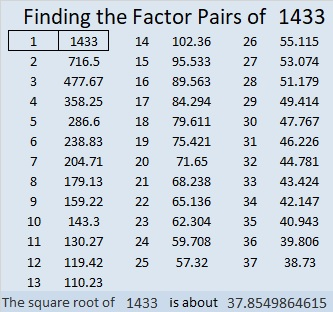# 1433 and Level 2

There is only one way to arrange the numbers from 1 to 10 in both the first column and the top row to make this puzzle function like a multiplication table. Can you find that one way?Print the puzzles or type the solution in this excel file:  10 Factors 1432-1442

The puzzle number is 1433. Here are some facts about that number:

• 1433 is a prime number.
• Prime factorization: 1433 is prime.
• 1433 has no exponents greater than 1 in its prime factorization, so √1433 cannot be simplified.
• The exponent in the prime factorization is 1. Adding one to that exponent we get (1 + 1) = 2. Therefore 1433 has exactly 2 factors.
• The factors of 1433 are outlined with their factor pair partners in the graphic below.

How do we know that 1433 is a prime number? If 1433 were not a prime number, then it would be divisible by at least one prime number less than or equal to √1433. Since 1433 cannot be divided evenly by 2, 3, 5, 7, 11, 13, 17, 19, 23, 29, 31, or 37, we know that 1433 is a prime number.1433 is the sum of two squares:
37² + 8² = 1433

1433 is the hypotenuse of a Pythagorean triple:
592-1305-1433 calculated from 2(37)(8), 37² – 8², 37² + 8²

Here’s another way we know that 1433 is a prime number: Since its last two digits divided by 4 leave a remainder of 1, and 37² + 8² = 1433 with 37 and 8 having no common prime factors, 1433 will be prime unless it is divisible by a prime number Pythagorean triple hypotenuse less than or equal to √1433. Since 1433 is not divisible by 5, 13, 17, 29, or 37, we know that 1433 is a prime number.

This site uses Akismet to reduce spam. Learn how your comment data is processed.